This math fractions ClipArt gallery offers 18 illustrations of a circle, or pie, broken into quarter or fourth slices. This includes images of individual slices, as well as the pie with missing slices.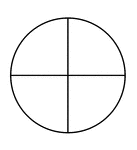### Fraction Pie Divided into Quarters

A circle divided into quarters.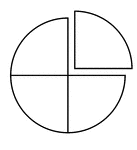### Fraction Pie Divided into Quarters

A circle divided into quarters with one quarter separated.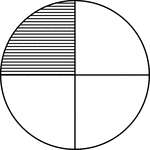### Fraction Pie Divided into Quarters

A circle divided into quarters with one quarter shaded.### Fraction Pie Divided into Quarters

A circle divided into quarters with two quarters shaded.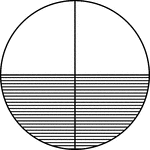### Fraction Pie Divided into Quarters

A circle divided into quarters with two quarters shaded.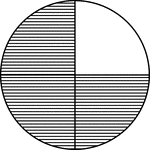### Fraction Pie Divided into Quarters

A circle divided into quarters with three quarters shaded.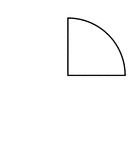### One Quarter of a Fraction Pie

One quarter of a circle.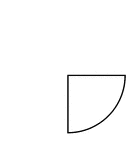### One Quarter of a Fraction Pie

One quarter of a circle.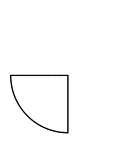### One Quarter of a Fraction Pie

One quarter of a circle.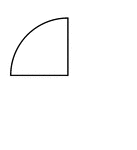### One Quarter of a Fraction Pie

One quarter of a circle.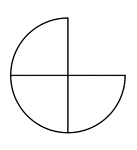### Three Quarters of a Fraction Pie

Three quarters of a circle.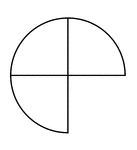### Three Quarters of a Fraction Pie

Three quarters of a circle.### Three Quarters of a Fraction Pie

Three quarters of a circle.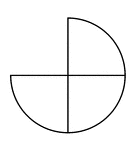### Three Quarters of a Fraction Pie

Three quarters of a circle.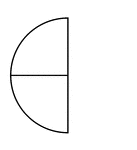### Two Quarters of a Fraction Pie

Two quarters of a circle.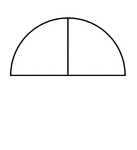### Two Quarters of a Fraction Pie

Two quarters of a circle.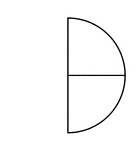### Two Quarters of a Fraction Pie

Two quarters of a circle.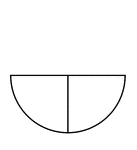### Two Quarters of a Fraction Pie

Two quarters of a circle.